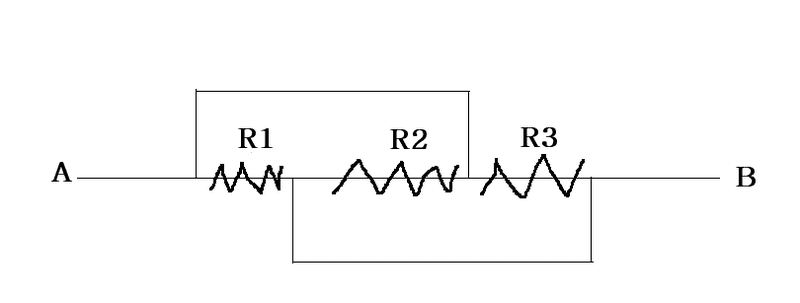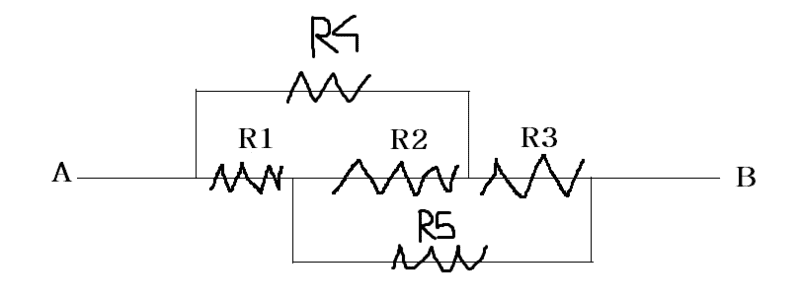# Finding equivalent resistance in complex circuit

In my previous question , situated here, I asked about finding the equivalent resistance of the following circuit :I got some very good answers and some tips. Now what if the same circuit is modified by adding two more resistors on the free connecting wires like this :How should I find the equivalent resistance now ?
I probably can't redraw the circuit as mentioned in answers to my previous question since every wire has a resistor on it.